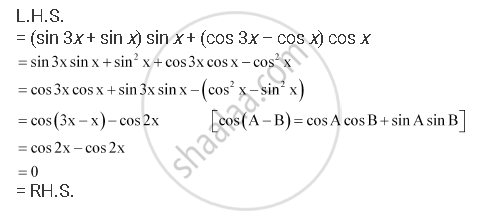CBSE (Arts) Class 11CBSE
Share

# Prove That: (Sin 3x + Sin X) Sin X + (Cos 3x – Cos X) Cos X = 0 - CBSE (Arts) Class 11 - Mathematics

ConceptSigns of Trigonometric Functions

#### Question

Prove that: (sin 3+ sin x) sin + (cos 3– cos x) cos = 0

#### SolutionIs there an error in this question or solution?

#### APPEARS IN

NCERT Solution for Mathematics Textbook for Class 11 (2018 to Current)
Chapter 3: Trigonometric Functions
Q: 2 | Page no. 81

#### Video TutorialsVIEW ALL 

Solution Prove That: (Sin 3x + Sin X) Sin X + (Cos 3x – Cos X) Cos X = 0 Concept: Signs of Trigonometric Functions.
S